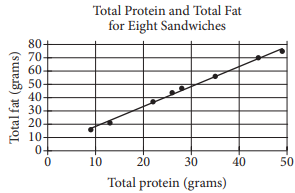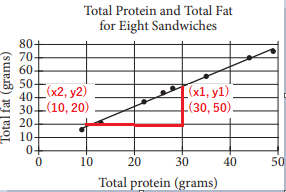mycollegehive
The scatterplot above shows the numbers of grams of both t...
SAT-math-test SAT math0The scatterplot above shows the numbers of grams of both total protein and total fat for eight sandwiches on a restaurant menu. The line of best fit for the data is also shown. According to the line of best fit, which of the following is closest to the predicted increase in total fat, in grams, for every increase of 1 gram in total protein?

A) 2.5

B) 2.0

C) 1.5

D) 1.0

1.6K viewsShareFollowUniversity of Benin Nigeria
30 May 2020University of Lagos Nigeria
12 August 20200The predicted increase in total fat, in grams, for every increase of 1 gram in total protein is represented by the slope of the line of best fit

slope = (y2 - y1) / (x2 - x1)slope = (20 - 50) / (10 - 30)

= -30 / -20

= 1.5

Ans CShare

### Groups

How to insert math formulas/equations### Related Tags

SAT-math-test

14 followers

476 questionsSAT

9 followers

487 questionsmath

23 followers

1262 questions